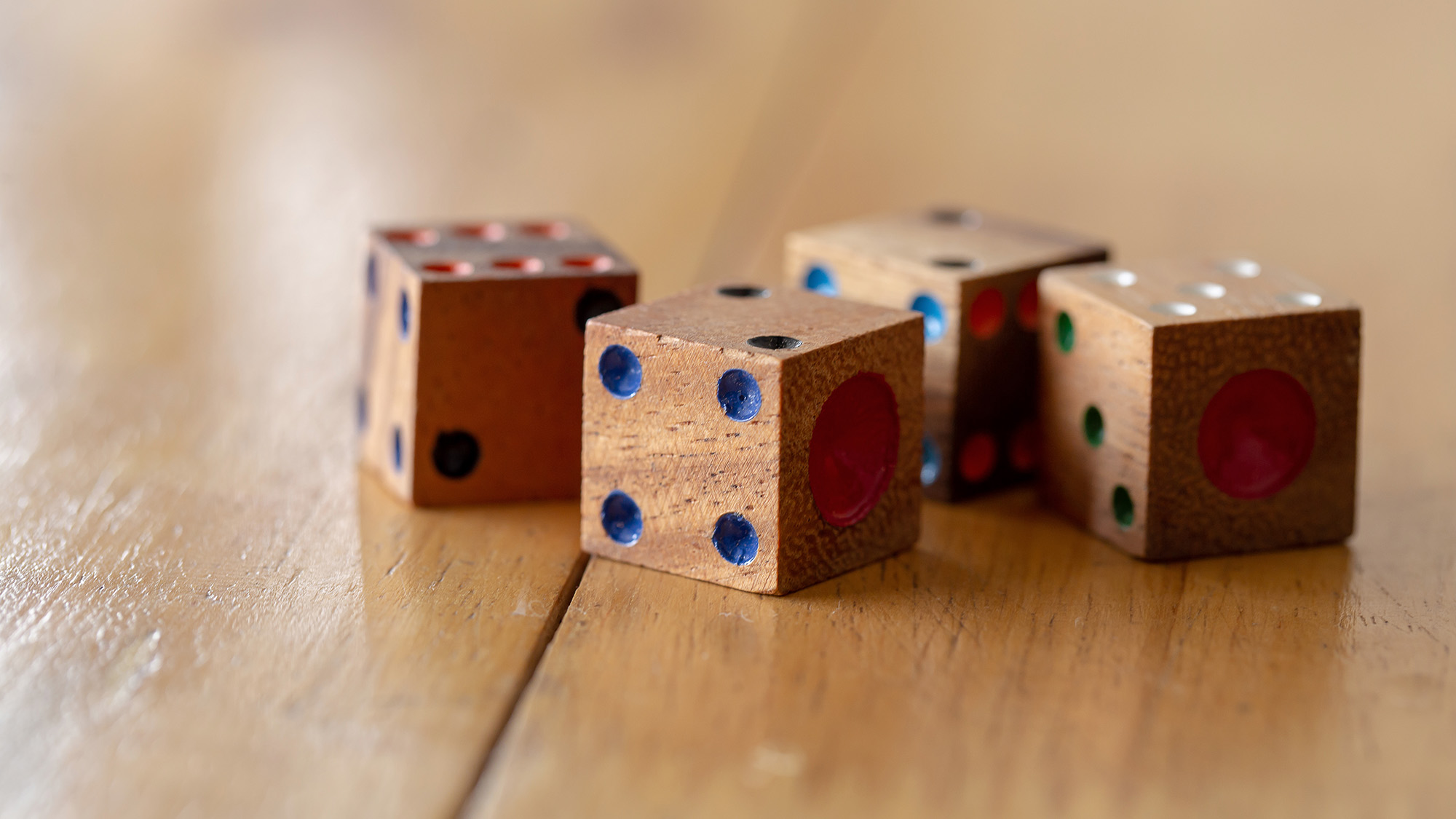## Learning Packs##### 00a. Family Dice Games##### 00b. Family Card Games##### 01a. Add 1 2 3##### 01b. Take 1 2 3##### 02. Doubles and Near Doubles##### 03a. Number Bonds Card Games##### 03b. Number Bonds Dice Games##### 04a. Addition up to total 12 - Dice##### 04b. Addition up to total 12 - Dominoes##### 05. Subtraction - max diff 9##### 06. Teens##### 07. Bridging through 10##### 08a. Addition up to total 20##### 08b. Subtraction - max diff 18##### 09. Tens and Units##### 10. Add and take 10, 9 is almost 10##### 11. Add and Subtract up to total 100##### 12. HTU##### 13. Further Place Value Games##### 14. Mental Arithmetic Games##### 15. Checking and Estimating##### 16. Times Tables - card games##### 17. Times Tables - dice games##### 18. Times Tables - other games##### 19. Understanding Fractions##### 20. Make Whole Fraction Games##### 21. Introduction to Mixed and Improper Fractions##### 22. Equivalent Fractions##### 23. Add & Subtract Fractions